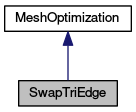MeshKit  1.0
SwapTriEdge Class Reference

`#include <SwapTriEdge.hpp>`

Inheritance diagram for SwapTriEdge:[legend]

List of all members.

struct  FlipEdge

## Public Member Functions

SwapTriEdge (Mesh *m, double angle=10.0)
Constructor ...
~SwapTriEdge ()
void setCreaseAngle (double a)
void setConstraintEdges (vector< Edge * > &edges)
size_t get_number_of_edges_flipped () const
int apply_rule (int r=DELAUNAY_RULE)

## Static Public Attributes

static const int DELAUNAY_RULE = 0
static const int DEGREE_REDUCTION_RULE = 1
static const int ADVANCE_FRONT_RULE = 2

## Private Member Functions

bool isIdeal (const Vertex *v) const
bool unchecked (const Face *f) const
int one_sweep (int entity, int r)
int atomicOp (const Face *face, int r)
int atomicOp (Vertex *v, int r)
virtual int commit (const FlipEdge &edge)
virtual bool is_edge_flip_allowed (const FlipEdge &edge, int r) const

## Private Attributes

Meshmesh
double creaseAngle
size_t num_edges_flipped

## Detailed Description

Definition at line 8 of file SwapTriEdge.hpp.

## Constructor & Destructor Documentation

 SwapTriEdge ( Mesh * m, double angle = `10.0` ) ` [inline]`

Constructor ...

Definition at line 17 of file SwapTriEdge.hpp.

 ~SwapTriEdge ( ) ` [inline]`

Definition at line 22 of file SwapTriEdge.hpp.

## Member Function Documentation

 int apply_advance_front_rule ( ) ` [private]`
 int apply_rule ( int r = `DELAUNAY_RULE` )
 int atomicOp ( const Face * face, int r ) ` [private]`
 int atomicOp ( Vertex * v, int r ) ` [private]`
 virtual int commit ( const FlipEdge & edge ) ` [private, virtual]`
 size_t get_number_of_edges_flipped ( ) const` [inline]`

Definition at line 33 of file SwapTriEdge.hpp.

 virtual bool is_edge_flip_allowed ( const FlipEdge & edge, int r ) const` [private, virtual]`
 bool isIdeal ( const Vertex * v ) const` [inline, private]`

Definition at line 46 of file SwapTriEdge.hpp.

 int one_sweep ( int entity, int r ) ` [private]`
 void setConstraintEdges ( vector< Edge * > & edges ) ` [inline]`

Definition at line 29 of file SwapTriEdge.hpp.

 void setCreaseAngle ( double a ) ` [inline]`

Definition at line 25 of file SwapTriEdge.hpp.

 bool unchecked ( const Face * f ) const` [inline, private]`

Definition at line 53 of file SwapTriEdge.hpp.

## Member Data Documentation

 const int ADVANCE_FRONT_RULE = 2` [static]`

Definition at line 13 of file SwapTriEdge.hpp.

 double creaseAngle` [private]`

Definition at line 41 of file SwapTriEdge.hpp.

 const int DEGREE_REDUCTION_RULE = 1` [static]`

Definition at line 12 of file SwapTriEdge.hpp.

 const int DELAUNAY_RULE = 0` [static]`

Definition at line 11 of file SwapTriEdge.hpp.

 Mesh* mesh` [private]`

Definition at line 40 of file SwapTriEdge.hpp.

 size_t num_edges_flipped` [private]`

Definition at line 42 of file SwapTriEdge.hpp.

The documentation for this class was generated from the following file: